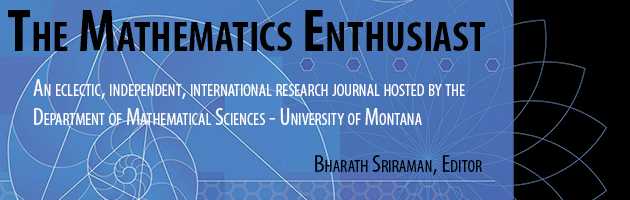•
•#### Article Title

Justifying Euler's formula through motion in a plane

15

3

#### Abstract

In this paper we consider motion of an object in a plane to provide a mechanical interpretation of Euler's formula. We believe that this approach contributes to a deeper and more intuitive understanding of Euler's formula and can especially be useful for students learning it and encountering complex numbers for the first time. Euler's formula can be introduced in many ways and using various approaches, but not all of these approaches help develop an intuition for where it comes from and why it works. We believe that the emphasis on physical interpretations and connections to motion can contribute to a more natural introduction and easier understanding of this fascinating formula. In this paper, we describe and consider motion in a plane, using it to give a detailed explanation of Euler's formula. Also, our paper points to the need for an integrated approach to teaching mathematics and physics. In our opinion the interpretation of mathematical results based on the physical phenomena and processes has an important methodological and motivational role in the process of learning. We believe students will be more successful in using this and other formulas of mathematics if they understand them first.

397

406

COinS